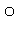# AUTOSYM

AUTOSYM is an alternative way of specifying symmetry. When AUTOSYM is present, symmetry data is generated. This data is similar to that supplied by the user when SYMMETRY is used. The 'rules' AUTOSYM uses are these:

• All bond-lengths which are within 0.0001Å are set equal.
• All bond-angles which are within 0.0057are set equal.
• All dihedral angles which are within 0.0057are set equal.
• All dihedral angles which are within 0.0057of the negative of an existing dihedral are set equal to the negative of the existing dihedral.

These 'rules' are a re-wording of internal SYMMETRY functions 1, 2, 3, and 14. The .arc file will include the symmetry data. In order to change the .arc file symmetry data into normal symmetry data, change AUTOSYM in the .arc file to SYMMETRY.

AUTOSYM only adds symmetry to coordinates that can be optimized.  If a coordinate has an optimization flag of "0", it will not be looked at by AUTOSYM.

Consider benzene:  If someone built the Z-matrix in internal coordinates, they would likely mark the C-C and C-H distances as optimizable, and lock the angles and dihedrals.  If they used AUTOSYM, then it would symmetry-relate all the C-C distances and all of the C-H distances, but leave the angles and dihedrals untouched.